# 【C#本质论 十】合式类型（一）重写Object成员及操作符重载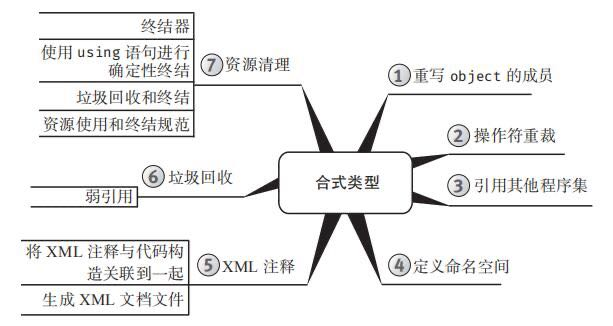## 重写Object的成员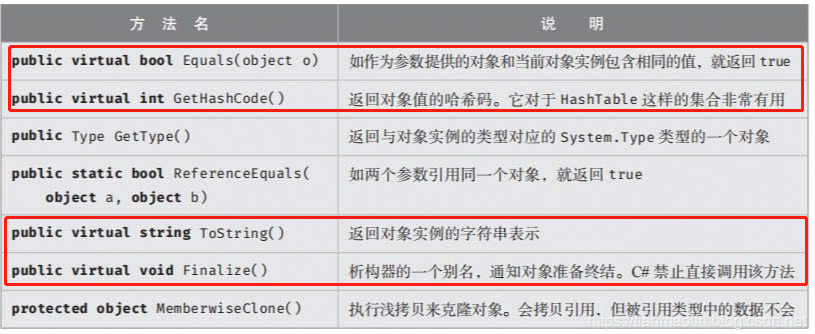### 重写ToSring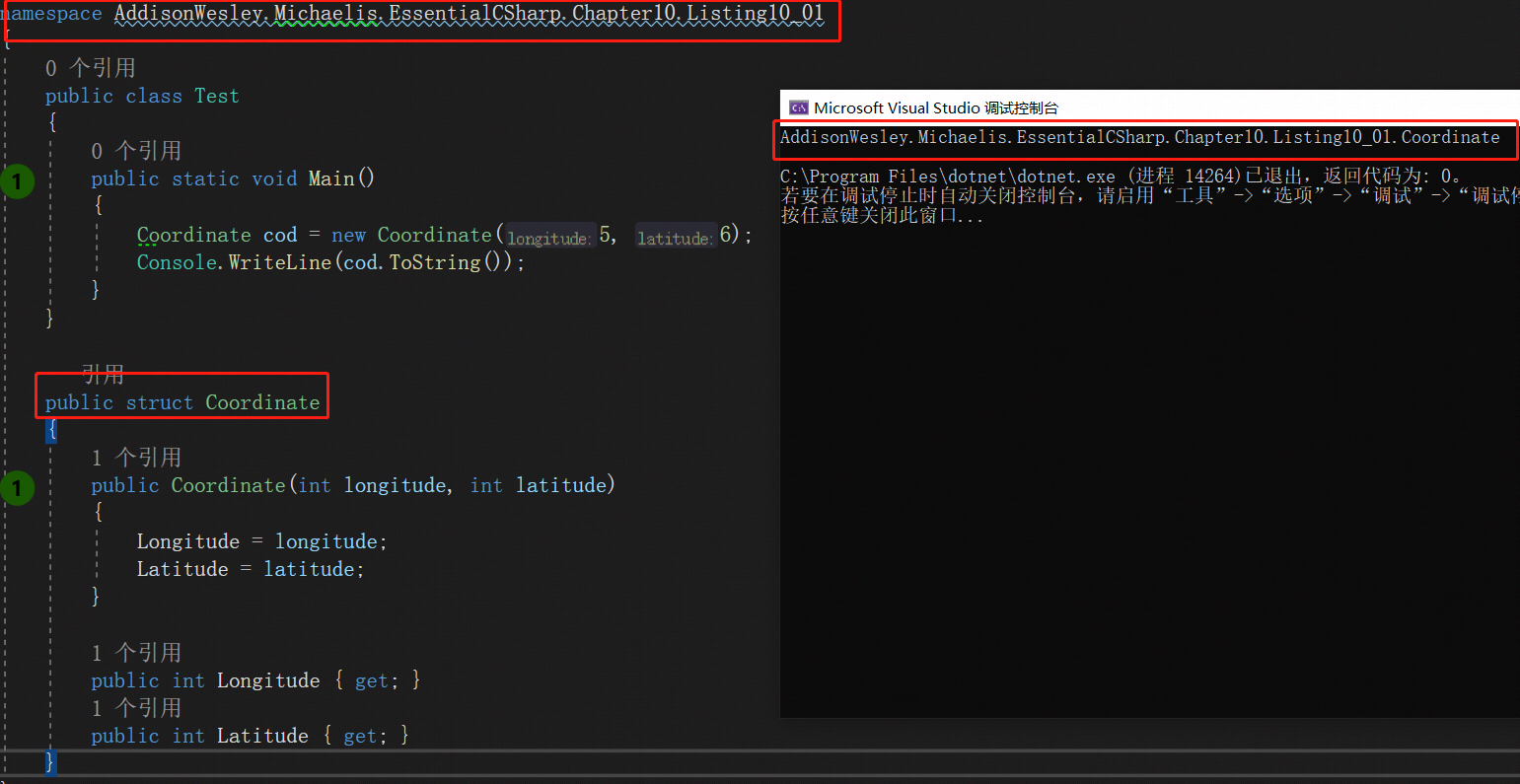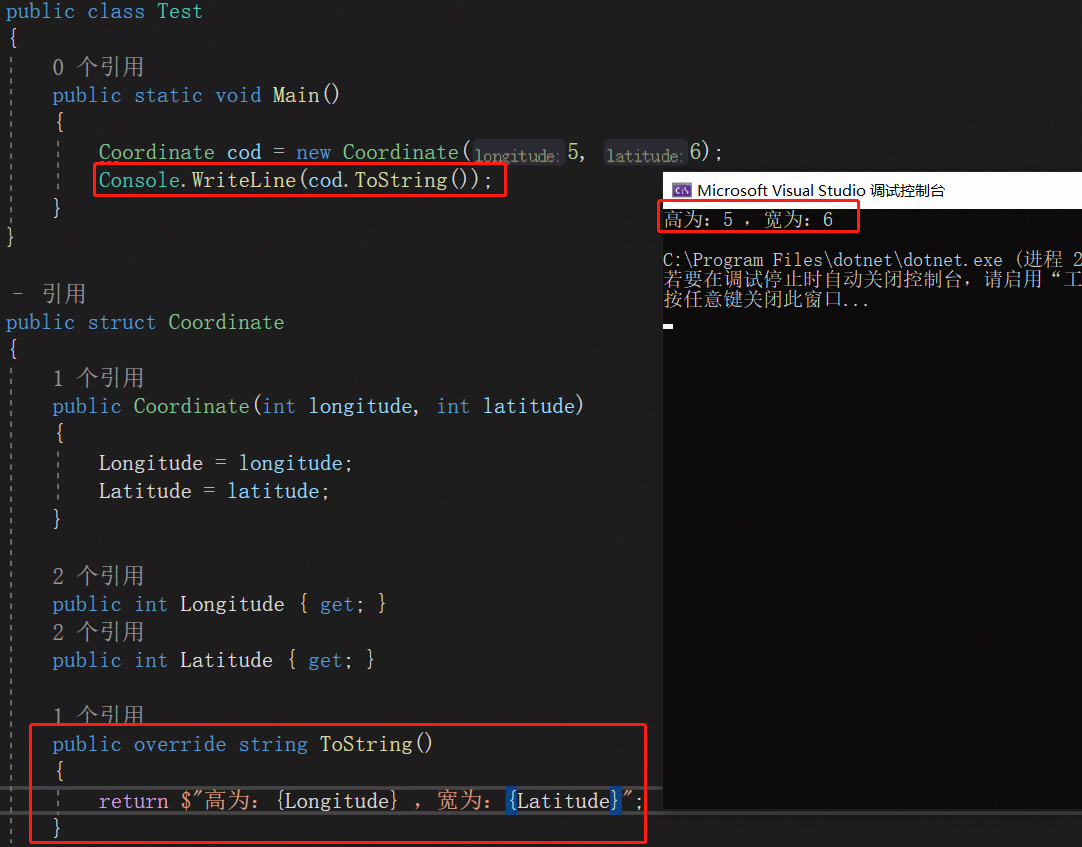• 如需返回有用的、面向开发人员的诊断字符串，就要重写ToString（）
• 要使ToString（）返回的字符串简短。
• 不要从ToString（）返回空字符串来代表“空”（null）。
• 避免ToString（）引发异常或造成可观察到的副作用（改变对象状态）
• 如果返回值与语言文化相关或要求格式化（例如DateTime），就要重载ToString（string format）或实现IFormattable。
• 考虑从ToString（）返回独一无二的字符串以标识对象实例。

### 重写GetHashCode()

GetHashCode方法是用来获取和对象对应的哈希码，有两种情况必须重写该方法：

• 重写Equals()方法一定要重写GetHashCode()，否则编译器会有警告
• 将类作为hash表集合的键使用的时候也要重写GetHashCode()。

）。

• 必须相等的对象必然有相等的哈希码（若a.Equals（b），则a.GetHashCode（）==b.GetHashCode（））。也就是相等的哈希码是对象相等性的必要不充分条件，对象相等则哈希码一定相等，哈希码相等对象不一定相等，还需要其它条件来满足
• 必须：在特定对象的生存期内，GetHashCode（）始终返回相同的值，即使对象的数据发生了改变。许多时候应缓存方法的返回值，从而确保这一点。
• 必须GetHashCode（）不应引发任何异常；GetHashCode（）总是成功返回一个值。
• 性能哈希码应尽可能唯一。但由于哈希码只是返回一个int，所以只要一种对象包含的值比一个int能够容纳得多（这就几乎涵盖所有类型了），那么哈希码肯定存在重复。一个很容易想到的例子是long，因为long的取值范围大于int，所以假如规定每个int值都只能标识一个不同的long值，那么肯定剩下大量long值没法标识。
• 性能可能的哈希码值应当在int的范围内平均分布。例如，创建哈希码时如果没有考虑到字符串在拉丁语言中的分布主要集中在初始的128个ASCII字符上，就会造成字符串值的分布非常不平均，所以不能算是好的GetHashCode（）算法。
• 性能：GetHashCode（）的性能应该优化。GetHashCode（）通常在Equals（）实现中用于“短路”一次完整的相等性比较（哈希码都不同，自然没必要进行完整的相等性比较了）。所以，当类型作为字典集合中的键类型使用时，会频繁调用该方法
• 性能两个对象的细微差异应造成哈希值的极大差异。理想情况下，1 bit的差异应造成哈希码平均16 bits的差异。这有助于确保不管哈希表如何对哈希值进行“装桶”（bucketing），也能保持良好的平衡性。
• 安全性：攻击者应难以伪造具有特定哈希码的对象。攻击手法是向哈希表中填写大量哈希为同一个值的数据。如哈希表的实现不高效，就易于受到DOS（拒绝服务）攻击。

namespace AddisonWesley.Michaelis.EssentialCSharp.Chapter10.Listing10_02
{
public struct Coordinate
{
public Coordinate(Longitude longitude, Latitude latitude)
{
Longitude = longitude;
Latitude = latitude;
}

public Longitude Longitude { get; }
public Latitude Latitude { get; }

public override int GetHashCode()
{
int hashCode = Longitude.GetHashCode();
// As long as the hash codes are not equal
if(Longitude.GetHashCode() != Latitude.GetHashCode())
{
hashCode ^= Latitude.GetHashCode();  // eXclusive OR
}
return hashCode;
}

public override string ToString()
{
return string.Format("{0} {1}", Longitude, Latitude);
}

}

public struct Longitude { }
public struct Latitude { }
}


• 首先向来自相关类型的哈希码应用XOR操作符，并确保操作数不相近或相等（否则结果全零）

### 重写Equals()

• 两个引用假如引用同一个实例，就说这两个引用是同一的。object（因而延展到所有派生类型）提供名为**ReferenceEquals（）**的静态方法来显式检查对象同一性
• 引用同一性只是“相等性”的一个例子。两个对象实例的成员值部分或全部相等，也可以说它们相等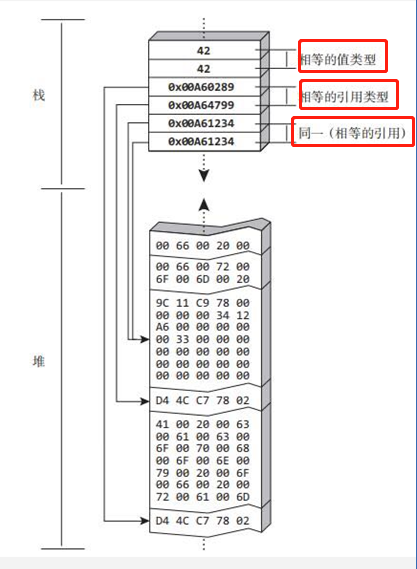public class Program
{
public static void Main()
{
TML tml1 = new TML("PV", "1000", "09187234");
TML tml2 = tml1;
TML tml3 = new TML("PV", "1000", "09187234");

// 对象是不是引用同一
if (!TML.ReferenceEquals(tml1, tml2))
{
throw new Exception("serialNumber1 does NOT " + "reference equal serialNumber2");
}
// 不引用同一总相等吧
else if (!tml1.Equals(tml2))
{
throw new Exception("serialNumber1 does NOT equal serialNumber2");
}
else
{
Console.WriteLine(
"serialNumber1 reference equals serialNumber2");
Console.WriteLine(
"serialNumber1 equals serialNumber2");
}

// 对象是不是引用同一
if (TML.ReferenceEquals(tml1, tml3))
{
throw new Exception("serialNumber1 DOES reference " + "equal serialNumber3");
}
// 不引用同一总相等吧
else if (!tml1.Equals(tml3) ||tml1!= tml3)
{
throw new Exception("serialNumber1 does NOT equal serialNumber3");
}

Console.WriteLine("serialNumber1 equals serialNumber3");
}
}

public class TML
{
public TML(string name, string price, string number)
{
Name = name;
Price = price;
Number = number;
}

public string Name { get; }
public string Price { get; }

public string Number { get; }
}


serialNumber1 reference equals serialNumber2
serialNumber1 equals serialNumber2
serialNumber1 equals serialNumber3


• 只有引用类型才能使用ReferenceEquals方法判断，值类型的调用永远是false，因为值类型要调用该方法一定要装箱为object，而各自装箱产生的引用一定不是同一个。
• Object.Equals（）的实现只是简单调用了一下ReferenceEquals方法，所以用处很有限。大多数情况下需要重写。

1. 检查是否为null--------不为null才能继续哦，否则没有比较的必要
2. 如果是引用类型，就检查引用是否相等------引用同一则一定相等
3. 检查数据类型是否相同
4. 调用一个指定了具体类型的辅助方法，它的操作数是具体要比较的类型而不是object（例如代码清单10.5中的Equals（Coordinate obj）方法）
5. 可能要检查哈希码是否相等来短路一次全面的、逐字段的比较---------相等的两个对象不可能哈希码不同，哈希码不同则一定不等,但是有时候散列不均匀或者没有缓存，则可能导致返回的hash值并非独一无二，所以不能依赖它判断亮哥对象是否相等。
6. 如基类重写了Equals（），就检查base.Equals（）
7. 比较每一个标识字段（关键字段），判断是否相等
8. 重写GetHashCode（）
9. 重写==和！=操作符（参见下一节）

namespace AddisonWesley.Michaelis.EssentialCSharp.Chapter10.Listing10_05
{
using System;

public class Program
{
public static void Main()
{
//...

Coordinate coordinate1 =
new Coordinate(new Longitude(48, 52),
new Latitude(-2, -20));

// Value types will never be reference equal
if(Coordinate.ReferenceEquals(coordinate1,
coordinate1))
{
throw new Exception(
"coordinate1 reference equals coordinate1");
}

Console.WriteLine(
"coordinate1 does NOT reference equal itself");
}
}

public struct Coordinate : IEquatable<Coordinate>
{
public Coordinate(Longitude longitude, Latitude latitude)
{
Longitude = longitude;
Latitude = latitude;
}

public Longitude Longitude { get; }
public Latitude Latitude { get; }

public override bool Equals(object obj)
{
// STEP 1: Check for null
if (obj == null)
{
return false;
}
// STEP 3: Equivalent data types
if (this.GetType() != obj.GetType())
{
return false;
}
return Equals((Coordinate)obj);
}

public bool Equals(Coordinate obj)
{
// STEP 1: Check for null if a reference type
// (e.g., a reference type)
// if (obj == null)
// {
//     return false;
// }

// STEP 2: Check for ReferenceEquals if this
// is a reference type.
// if ( ReferenceEquals(this, obj))
// {
//   return true;
// }

// STEP 4: Possibly check for equivalent hash codes.
// if (this.GetHashCode() != obj.GetHashCode())
// {
//    return false;
// }

// STEP 5: Check base.Equals if base overrides Equals().
// System.Diagnostics.Debug.Assert(
//     base.GetType() != typeof(object) );
// if ( !base.Equals(obj) )
// {
//    return false;
// }

// STEP 6: Compare identifying fields for equality
// using an overload of Equals on Longitude
return ((Longitude.Equals(obj.Longitude)) &&
(Latitude.Equals(obj.Latitude)));
}

// STEP 7: Override GetHashCode
public override int GetHashCode()
{
int hashCode = Longitude.GetHashCode();
hashCode ^= Latitude.GetHashCode(); // Xor (eXclusive OR)
return hashCode;
}
public static bool operator ==(
Coordinate leftHandSide,
Coordinate rightHandSide)
{
return (leftHandSide.Equals(rightHandSide));
}

public static bool operator !=(
Coordinate leftHandSide,
Coordinate rightHandSide)
{
return !(leftHandSide.Equals(rightHandSide));
}
}

public struct Longitude
{
public Longitude(int x, int y) { }
}

public struct Latitude
{
public Latitude(int x, int y) { }
}
}


• 要一起实现GetHashCode（）、Equals（）、==操作符和！=操作符，缺一不可
• 要用相同算法实现Equals（）、==和！=
• 避免在GetHashCode（）、Equals（）、==和！=的实现中引发异常
• 避免在可变引用类型（也就是Object）上重载相等性操作符（如重载的实现速度过慢，也不要重载）。
• 要在实现IComparable时实现与相等性相关的所有方法。

### 用元组重写GetHashCode()和Equals()

    public class TML : IEquatable<TML>
{
public TML(string name, string price, string number)
{
Name = name;
Price = price;
Number = number;
}

public string Name { get; }
public string Price { get; }

public string Number { get; }

public bool Equals(TML tml)
{
return (Name, Price, Number).Equals((tml.Name, tml.Price, tml.Number));
}

public override int GetHashCode()
{
return (Name, Price, Number).GetHashCode();
}
}


## 操作符重载

• 赋值运算符=不支持重载
• 重载的操作符不能通过IntelliSense呈现，也就是不能智能提示。
• ==默认也只是执行引用相等性检查，所以为了保证和Equals的同一性，一定也要重写该操作符！

### ==和!=重载

 public sealed class ProductSerialNumber
{
public ProductSerialNumber(
string productSeries, int model, long id)
{
ProductSeries = productSeries;
Model = model;
Id = id;
}

public string ProductSeries { get; }
public int Model { get; }
public long Id { get; }

public bool Equals(ProductSerialNumber obj)
{
return ((obj != null) && (ProductSeries == obj.ProductSeries) && (Model == obj.Model) && (Id == obj.Id));
}

public static bool operator ==(
ProductSerialNumber leftHandSide,
ProductSerialNumber rightHandSide)
{
if (ReferenceEquals(leftHandSide, null))
{
return ReferenceEquals(rightHandSide, null);
}

return (leftHandSide.Equals(rightHandSide));
}

public static bool operator !=(
ProductSerialNumber leftHandSide,
ProductSerialNumber rightHandSide)
{
return !(leftHandSide == rightHandSide);
}
}


### +和-重载

 public struct Coordinate
{
public Coordinate(Longitude longitude, Latitude latitude)
{
Longitude = longitude;
Latitude = latitude;
}

public static Coordinate operator +(
Coordinate source, Arc arc)
{
Coordinate result = new Coordinate(
new Longitude(
source.Longitude + arc.LongitudeDifference),
new Latitude(
source.Latitude + arc.LatitudeDifference));
return result;
}

public static Coordinate operator -(
Coordinate source, Arc arc)
{
Coordinate result = new Coordinate(
new Longitude(
source.Longitude - arc.LongitudeDifference),
new Latitude(
source.Latitude - arc.LatitudeDifference));
return result;
}
}


### 转型操作符

 public struct Latitude
{
public Latitude(double decimalDegrees)
{
DecimalDegrees = Normalize(decimalDegrees);
}

public double DecimalDegrees { get; }

// ...

public static implicit operator double(Latitude latitude)
{
return latitude.DecimalDegrees;
}
public static implicit operator Latitude(double degrees)
{
return new Latitude(degrees);
}

private static double Normalize(double decimalDegrees)
{
// here you would normalize the data
return decimalDegrees;
}
}©️2019 CSDN 皮肤主题: 点我我会动 设计师: 上身试试